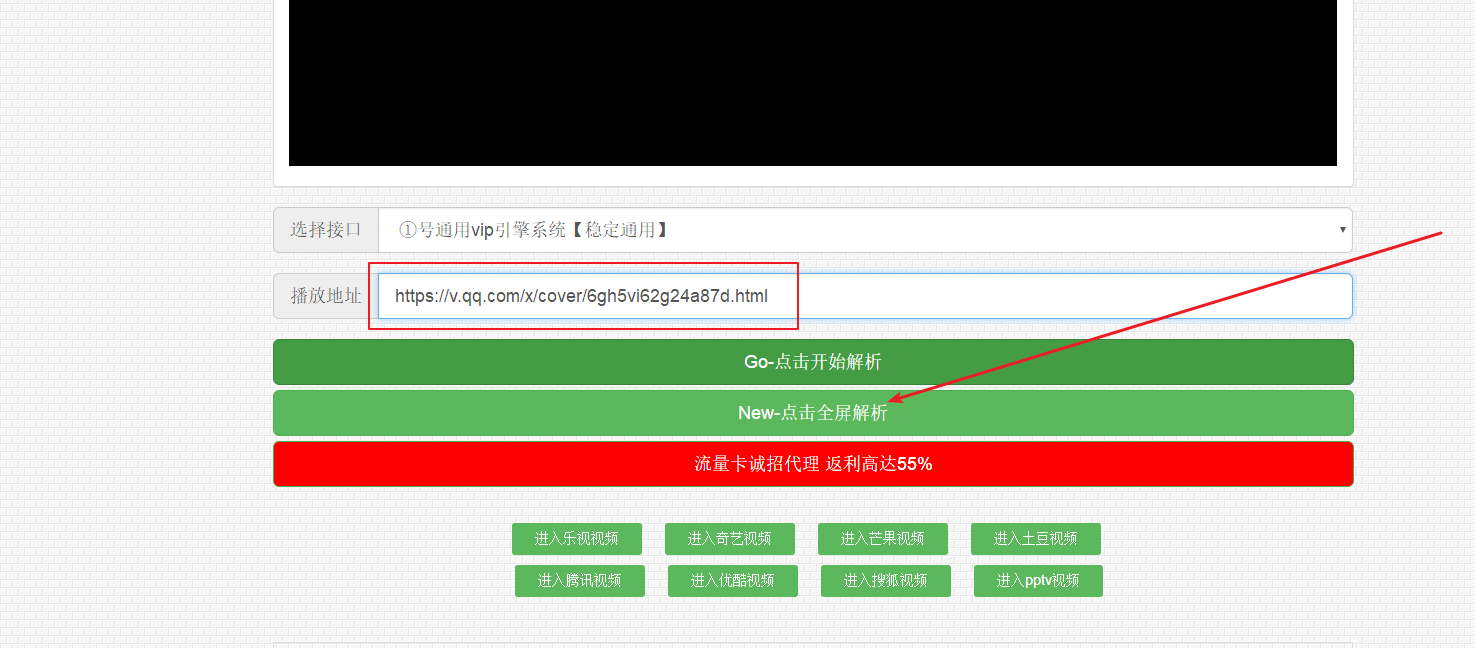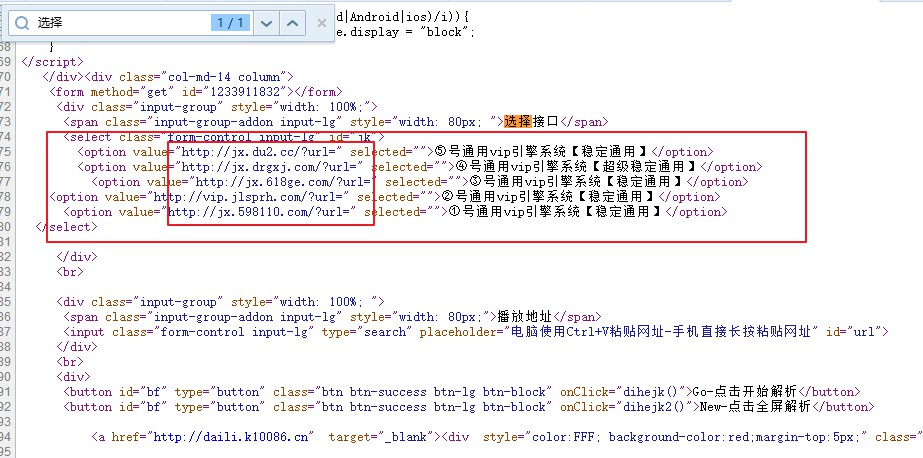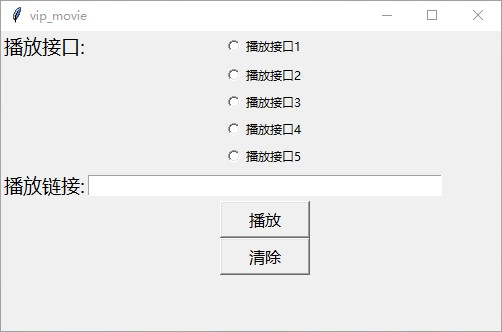零基础学python，制作全网Vip视频免费观看工具！ – Python量化投资

零基础学python，制作全网Vip视频免费观看工具！

• 　　python3.6.5
• 　　Windows

• 　　re
• 　　requests
• 　　tkinter
• 　　webbrowserresponse = requests.get('http://www.qmaile.com/')
response.encoding=response.apparent_encoding
reg=re.compile('<option value="(.*?)" selected="">')
res=re.findall(reg,response.text)
one=res
two=res
three=res
four=res
five=res

如果我们想做以下这款软件，5个接口单选按钮，随便选择一个，然后填上播放链接，最后点击播放，实现跳转到一个新网页，root = tk.Tk()
root.title('Vip电影播放_学习交流群：790921645')
root.geometry('500x250+100+100')# 宽*长
l1=tk.Label(root,text='播放接口:',font=("Arial",12),)#bg='pink'height=3
l1.grid(row=0,column=0)
l2=tk.Label(root,text='播放链接:',font=("Arial",12),)
l2.grid(row=6,column=0)
t1=tk.Entry(root,text='',width=50)
t1.grid(row=6,column=1)

#StringVar是Tk库内部定义的字符串变量类型，在这里用
# 于管理部件上面的字符；不过一般用在按钮button上
# 单选按钮
# 当用户选择某单选按钮时，同一组中的其他单选按钮不能同时选定。
# “这里有一组选项，您可以从中选择一个且只能选择一个。
var=tk.StringVar(value=None)
r1.grid(row=0,column=1,)
var.set(r1)
r2.grid(row=1,column=1)
r3.grid(row=2,column=1)
r4.grid(row=3,column=1)
r5.grid(row=4,column=1)

def play_movie():
webbrowser.open(var.get()+t1.get())

b1=tk.Button(root,text='播放',font=("Arial",12), width=8,command=play_movie)
b1.grid(row=7,column=1)

def del_text():
t1.delete(0,'end')

b2=tk.Button(root,text='清除',font=("Arial",12), width=8,command=del_text)
b2.grid(row=8,column=1)

root.mainloop()https://www.cnblogs.com/qdsn/p/11624583.html

「点点赞赏，手留余香」

还没有人赞赏，快来当第一个赞赏的人吧！
Python
Python
0 条回复 A 作者 M 管理员
所有的伟大，都源于一个勇敢的开始！